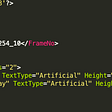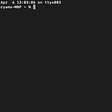# Problem Statement :

You are given a Binary search tree(BST) of integers and an integer ‘K’.

Your task is to find and return the sum of the first ‘K’ smallest elements of the BST.

The first line of input contains the elements of the tree in the level order form separated by a single space.

If any node does not have a left or right child, take -1 in its place.

The second line contains a single integer ‘K’.

Sample Input:

`8 4 12 1 6 -1 -1 -1 -1 -1 7 -1 -14`

Sample Output:

`18`

# Explanation of given Test Case :

For the given tree ‘K = 4’. The first ‘4’ smallest elements are 1, 4, 6, and 7. Sum of three smallest nodes in the BST = 1 + 4 + 6 + 7 = 18. Thus, you should return ‘18’ as the answer.

# Inorder Traversal Upto The First ‘K’ Nodes

While performing the inorder traversal of the BST recursively, along with ‘ROOT’, pass the integer ‘K’ as a reference. Whenever we visit the ‘ROOT’ during the inorder traversal, reduce the value of ‘K’ by one and add ‘ROOT->DATA’ to the sum of nodes until now. If at any point during the recursion, ‘K’ becomes ‘0’, we have visited the first ‘K’ nodes, so stop the recursion. The function ‘inorderTraversal’ computes the sum of the first ‘K’ smallest nodes in the BST using inorder traversal.

‘integer inorderTraversal(BinaryTreeNode ROOT, integer K)’:

• If ‘ROOT’ is equal to ‘NULL’, then ‘ROOT’ is not a valid node: Return 0, as the sum.
• If ‘K’ is equal to ‘0’, then we have already visited the first ‘K’ nodes: Return 0, as the sum.
• Set ‘SUM = inorderTraversal(ROOT->LEFT, K)’. This is the sum of the nodes in the left subtree of ‘ROOT’.
• If ‘K’ is equal to ‘0’, then we have already visited the first ‘K’ nodes while traversing the left subtree of ‘ROOT’: Return ‘SUM’, as the sum.
• ‘SUM += ROOT->DATA’. Add the data at ‘ROOT’ to the sum until now.
• ‘K-= 1’. As we have visited the ‘ROOT’ node, decrease ‘K’ by one.
• ‘SUM += inorderTraversal(ROOT->RIGHT, K)’. Add the sum of the nodes in the right subtree of ‘ROOT’ to ‘SUM’.
• Return ‘SUM’, as the sum of the first ‘K’ smallest nodes of the BST at ‘ROOT’.

## ALGORITHM:

• ‘SUM = inorderTraversal(ROOT, K)’. Compute the sum of the first ‘K’ smallest elements in the BST.
• Return ‘SUM’ as the answer.

Time Complexity : O(N)
Space Complexity : O(N)

# Code :

Placewit grows the best engineers by providing an interactive classroom experience and by helping them develop their skills and get placed in amazing companies.

--

--

--

## More from Placewit

Upskilling students for tech placements!

Love podcasts or audiobooks? Learn on the go with our new app.

## Custom links in Jaeger UI## How to Create Tfrecords from Partial Pascal VOC XML Annotation Format for Object Detection## Activate and Deactivate Your Virtual Environment.## Install Hyperledger Fabric on Debian 9## Web 1.0 Vs Web 2.0 Vs Web 3.0 Vs Web 4.0## Placewit

Upskilling students for tech placements!

## Longest Increasing Subsequence Coding Question## Koko Eating Bananas